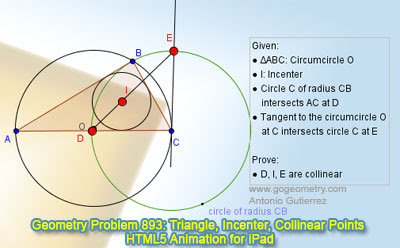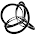## Wednesday, June 26, 2013

### Problem 893: Triangle, Circumcircle, Incenter, Circle, Tangent, Collinear Points

Geometry Problem. GeoGebra, HTML5 Animation for iPad and Nexus.

Level: Mathematics Education, High School, Honors Geometry, College.

Click the figure below to see the dynamic geometry demonstration of problem 892.1.Consider the line DE and try to prove that I lies on DE.
Let P be a point on CE on the other side of E.
Let ∠BAC be 2A and ∠DEC = X.
∠IBC = (1/2)∠ABC = (1/2)∠DCP = (1/2)*2*∠DEC = ∠DEC, so I,B,E,C concylic.
Therefore ∠BIE = ∠BCE = 2A.

Now consider ∠BDE = (1/2)∠BCE = (1/2)*2A = A.
Hence,
∠DBI = ∠DBC - ∠IBC
= ∠BDC - X
= ∠BDE + ∠EDC - X
= A + ∠DEC - X
= A + X - X
= A

Combining all the results, we have ∠DBI = A, ∠BDE = A, and ∠BIE = 2A
By the idea of exterior angle of triangle, D,I,E is collinear.

1.To William

Your statement " ∠IBC = (1/2)∠ABC = (1/2)∠DCP = (1/2)*2*∠DEC = ∠DEC, so I,B,E,C concylic." may not correct.
∠IBC =∠DEC doesn't imply I,B,E,C concylic .I,B,E,C concylic only if D, I, E are collinear .
Peter Tran

2.https://goo.gl/photos/Kmxu4USsFCZZEZXG8

Connect IC and IB
Triangle DIC congruent to BIC ..( case SAS)
So ∠ (IDC)= ∠ (IBC)= ∠ (B/2)
Triangle DCE is isosceles and ∠ (DCE)= ∠ (DCB)+ ∠ (BCE)= ∠ ( C) +∠ (A)= 180- ∠ (B)
So ∠ (CDE)=90-1/2. ∠ (DCE)= 90-(90-∠ (B)/2))= ∠ (B/2)
So ∠ (CDE)= ∠ (IDC) => D, I, E are collinear

3.Problem 893
Let DE intersects the bisector the angle ACB at point C.Is <BEK=<BED=<BAD/2=<KCB so KBEC is cyclic.But <KBC=<KEC=<DEC=90-<ECD/2=90-(<ECB+<BCA)/2=90-(<CAB+<BCA)/2=<ABC/2=<IBC.So the points K,I conside .Therefore the points D,I and E are
collinear.
APOSTOLIS MANOLOUDIS 4 HIGH SHCOOL OF KORYDALLOS PIRAEUS GREECE